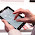### JavaScript Basics - Technoob Technology

Javascript is a Simple but very powerful programming language which was built with the evolution of technology. Javascript is used to build web pages, process data and as well as to create various applications. (mobile apps, desktop apps, games etc.)

Topics we gonna cover

• basic syntax
• variables and operators
• How to build a  simple calculator

.

Basic  Syntax

Javascript's basic syntax is very easy. Technology has driven hard programming languages into easy and simple languages. If you can understand the fundamentals you can keep learning this easily. It starts with the <script>  tag and ends with the </script> tag. All the codes go inside these tags.

Adding javascript to HTML pages is very easy. There are two different ways you could do that.

<script lanuage="javascript" type="text/javscript"> </script>

02)External Javascript

Adding the JS code inside the head tags is easy. But when you get familiar with Javascript and you type so many code lines, it will be so difficult to find the code lines and fix errors. In this case, you can use external javascript.

write your JS code in a text editor and save the file in .js extension

<script src="filename.js"></script>

NOTE: you have to include the path to the .js file if it is located in another folder.

TIP: Use various .js files for various functions. This will make it easy to fix bugs and rebuild codes.

Writing in the HTML document

We use ' document.write ' to write something inside the HTML page.

<script>
document.write("Hello world");
</script>

The output of the code

The symbol ";" is necessary after each code line. This helps the script to understand how to execute the code and if you forget to add this symbol it could lead to so many problems.

For a single line comment, you can use ' // 'at the beginning of the line.

//This is a single line comment

For a multiline comment use ' /* ' at the beginning of the comment and ' */ ' at the end of the line.

/* This is a multiline comment */

variables and operators

Variables and Operators are used to do different kind of arithmetic solutions and to do changes in HTML document.

Variables can be simply explained as memory packets. When you assign something as a variable, it is stored in the RAM. Assigning values to variables is easy.

var name = John;

This code simply stores a variable called name and assigns it value as John.

var name = John;
var age = 25;
var height = 125.6;
var married = true;

All of these are variables and there are different kind of values which can be assigned. String, Boolean, and integers are some of them. You can assign a name or a sentence as a string. A boolean value can be only assigned as true or false.

The most valuable thing in Javascript is it automatically understands the data type and assigns it. If you assign a name it stores it as a string and if you assign a number, it stores it as an integer.

var string = "Hello! Welcome!";
document.write(string);

The output of the code

This code will print 'Hello! Welcome!' into the HTML page.
NOTE: Javascript is a case-sensitive language which means String and string are two different variables.

Operators

There are some operators which are used to do match calculations in Javascript.

Math operators

-         Substraction
*   Multiplication
/ Division
++         Imcrement
-- Decrement

Assignment Operators

operator     Example    Equal to
=     x=y     x=y
+=     x+=y     x=x+y
-=     x-=y     x=x-y
/=     x/=y     x=x/y
*=     x*=y     x=x*y

Comparison Operators

Operator   Description

==   equal to
!=   not equal
>   greater than
=>   greater than or equal to
<   less than
=<         less than or equal to

Building a simple calculator using these operators and variables is easy. You can simply do this by using variables, math operators, assignment operators, and document.write command.

<script>

var a = 1;
var b = 2;
//enter the numbers you want.
var sum = a + b;
document.write(sum);

//multiplication
var c = 1;
var b = 2;
//enter the numbers you want.
var sum1 = c * b;
document.write(sum1);

//substraction
var e = 1;
var f = 2;
//enter the numbers you want.
var sum2 = e - f;
document.write(sum2);

</script>

The output of the code

HINT: you can use the + symbol with double qoutes to add text with the document.write commands

example: document.write(sum + " ,");
//if the sum = 20 this code outputs 20 ,

As this, you can build a simple calculator using these codes.

HINT: Try using alert command instead of document.write. This will give the output as an alert window.

example:         sum = 20;

Hope this guide could help you in understanding the basics of Javascript. Leave a suggestion or a comment to add or improve the user experience.

Popular Articles

How to speed up computer
Extend mobiles battery life

1.2.3.